Home CHEMISTRY CHEMISTRY FORM 2 TOPIC 7: CHEMICAL FORMULA BONDING AND NOMENCLATURE ~ CHEMISTRY FORM 2

# TOPIC 7: CHEMICAL FORMULA BONDING AND NOMENCLATURE ~ CHEMISTRY FORM 2

167
0
SHAREValency is the capacity of an atom to combine with one or more atoms to form a molecule or compound. Valency also refers the number of electrons that an atom can gain, lose or share in forming a chemical bond with another atom.
The valency (or combining power) depends on the number of electrons in the outermost orbit (or valency shell) involved in the formation of a chemical bond. The number of electrons in the valency shell is never greater than 7. The outermost electronic configuration is responsible for the variability of the valency.
Some elements exhibit more than one valency, i.e., they have variable valencies. Examples of elements with variable valencies are iron (2 and 3), tin (2 and 4) and copper (1 and 2). The other elements with variable valencies are as shown in table 7.1.
Valency and Oxidation States
There is a strong correlation between valency and oxidation state. The oxidation state of an element equals its valency or charge carried by its ion when an element ionizes in solution. An example of this relation is iron (II) whose oxidation state (or oxidation number) is 2 and its valency is 2.
The same case applies to iron (III). Other elements with variable valencies such as copper (I) and copper (II) have oxidation state equal to 1 and 2 respectively. The list continues. You will learn more about oxidation states later.
The valencies of the common transition elements should be memorized. Valencies of the normal elements may be deduced from the group number they occupy in the Periodic Table. The valencies of elements of group I to IV are equal to the group numbers they occupy in the periodic table.
The valency of an element in group V to VIII is equal to eight minus the group number. For example, the valency of chlorine which is in group VII is 1, i.e. (8 -7) =1. The valency of oxygen in group VI is 2, i.e. (8-6) =2. Elements in group 0 (or VIII) have zero valency i.e. (8 – 8) = 0.
Table 7.1. Valencies of common metals and non-metals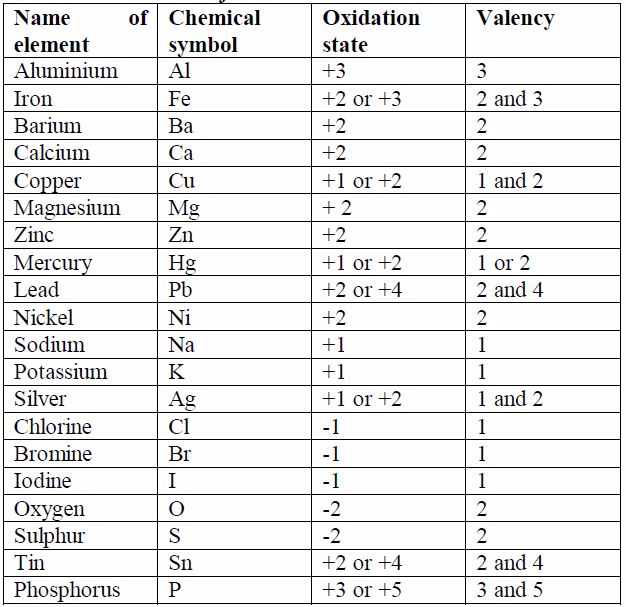Simple Formulae of Binary Compounds

Write simple formulae of binary compounds

#### Chemical Formulae

The Chemical formula is a method of representing the molecule of a compound by using chemical symbols. It is a way of expressing information about the atoms that constitute a particular chemical compound. The chemical formula identifies each constituent element by its chemical symbol and indicates the number of atoms of each element found in each single molecule of that compound.
The symbol for hydrogen atom is H. When two hydrogen atoms join together they form a molecule,H2. The number 2 to the right and below the symbol shows the number of atoms a molecule contains. P4 and S8 represents 4 atoms of phosphorus and 8 atoms of sulphur contained in one molecule of phosphorus and one molecule of sulphur respectively.
While the formula for chlorine molecule isCl2, it cannot be expressed as 2Cl. This is because 2Cl means two atoms of chlorine and not a molecule of chlorine.H 2 O stands for a molecule of water which consists of two hydrogen atoms and one oxygen atom.
H2SO4stands for a molecule of sulphuric acid containing 2atoms of hydrogen, 1 atom of sulphur and 4 atoms of oxygen.CaCO3 stands for a molecule of calcium carbonate containing 1atom of calcium, 1 atom of carbon and 3 atoms of oxygen.Where it deems necessary to show the number of molecules a compound contains, this is achieved by writing the appropriate number before the formula of the compound. A few examples are shown below:
• 2H 2O means two molecules of water
• 3H2 SO4 means three molecules of sulphuric acid
• 5CaCO3 means five molecules of calcium carbonate
It is important to note that the figure appearing before the formula multiplies the whole of it. For example, 3H2SO4 stands for 3 molecules of sulphuric acid containing six atoms of hydrogen, three atoms of sulphur and twelve atoms of oxygen.
It is a big mistake to think that the number 3 before the molecule multiplies only the symbol which immediately follows it (that is,H2). This is quite wrong. The 3 multiplies the whole of the formula

#### Formulae of Binary Compounds

A binary compound is a compound made of only two types of the reacting species, for example, sodium chloride (NaCl),which is made of only sodium and chlorine, is a binary compound. Look at table 7.1. The size of the charge on an ion isa measure of its valency or combining power.
You will notice that, ignoring the signs for the charge on ions, the value of the charge on ion is equal to the valency of the atom. You will need to memorise the valencies of these metals as much as possible so as to be able to write the formulae of their compounds correctly.
The following are the rules for writing down the chemicalformulae of chemical substances:
1. Metals (or their positively charged ions) must start in theformula, followed by non-metals (or their negatively chargedions).
2. Where the formula is to include a radical, the radical must betreated as a single atom and must be bracketed if need be.
3. Ammonium ion is to be treated as if it were a metal.
4. Positive charges must be equal to negative charges for aneutral molecule or compound.
5. Single elements; say Na, K, Si, Ag, etc. should not bebracketed.
6. Valencies of metals (or positive ions) should be exchangedand written as subscripts.
7. The valency of 1 is simply assumed and not written in theformula.
This is best shown by using some examples. The following procedure must be followed when writing the formulae of binary compounds:
1. Write down correct symbols for atoms of elements or ions that make up the compound.
2. Write down the valencies of the atoms of the elements.
3. Interchange the valencies and write them as subscripts in the final formula of the compound. Remember that the valency of 1 is not expressed in the formula. At this final step, the radicals must be bracketed if necessary.
Study the following examples and make sure you understand how this works:
Write the formula for aluminium oxide: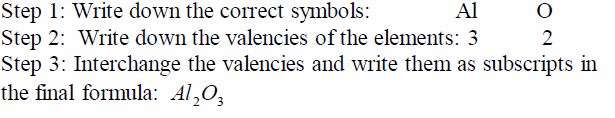Write down the formula for water: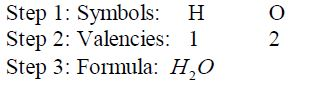Formula for sodium nitrate: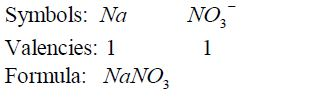Formula for calcium nitrate: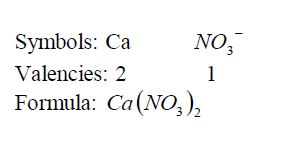Formula for ammonium nitrate: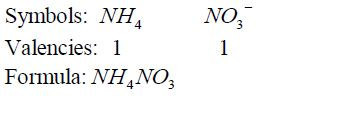Formula for calcium oxide: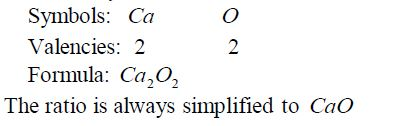Formula for aluminium sulphate: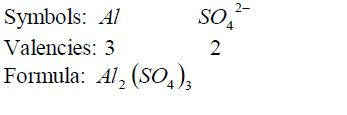Ammonia: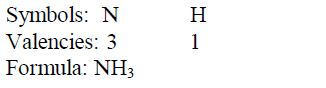Carbon dioxide: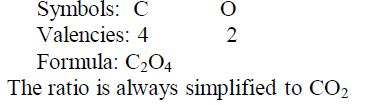#### Nomenclature of Inorganic Compounds

The term “nomenclature” refers to “system of naming”. The system of naming in use is that recommended by the IUPAC (International Union of Pure and Applied Chemistry). The modern system of naming reveals the type of elements present in a given compound. The old or trivial names have been dropped out.
Some common and important compounds have historical names that do not seem to fit in the system, for example water H O 2 ,ammonia 〈NH3〉, methane , CH4and mineral acids such as sulphuric (VI) acid〈H2SO4〉, nitric (V) acid〈HNO3〉and hydrochloric acid〈HCl〉. Also organic acids such as ethanoic acid (CH3COOH) are also included in this group. These names are trivial but they have been adopted in modern nomenclature.

#### If these exceptions are omitted, there are basic generalizations that are useful:

1. If there is a metal in the compound, it must be named first. In this case ammonium ion, NH4¹, is regarded as if it were a metal in the compounds it occurs such as NH4 NO3 , NH Cl 4 , etc.
2. For elements with variable valencies such as iron and lead,Roman numerals are included in the name to indicate the valency of the metal or the ion which is present. For example,iron (III) chloride contains Fe3+ while iron (II) chloride contains Fe² . The same case applies to lead (II) and lead (IV)compounds and so on.
3. Compounds containing two elements only (binary compounds) have names ending in …..ide; for example sodium chloride (NaCl), calcium bromide (CaBr2), magnesium nitrite( Mg3 N2) , etc. The important exception to this is hydroxides,which contains the hydroxide (OH) ion.
4. Compounds containing a poly atomic ion (usually containing oxygen) have names that end with …ate; for example calcium carbonate (CaCO3) , potassium nitrate(KNO3) , magnesium sulphate(MgSO4) , sodium ethanoate (CH3 COONa) , etc.
5. The names of some compounds use prefixes to tell you the number of that particular atom in the molecule. This is useful if two elements form more than one compound. For example:carbon monoxide (CO), carbon dioxide (CO2), nitrogen dioxide NO2 dinitrogen tetra oxide N2 O4 , sulphur dioxide SO2 sulphur trioxide SO3 , etc.
The following prefixes indicate the number of atoms in caseslike this: mono – one; di – two; tri – three; tetra – four; pent –five; hex – six; hept – seven; oct – eight; non – nine; and dec –ten.
###### The Concept of Empirical and Molecular Formulae
Explain the concept of empirical and molecular formulae
The empirical formula is the simplest formula of any compound.It expresses the simplest ratio of all the atoms or ions that makeup a certain compound.
For example, the empirical formula ofthe compound with the formula, C2H4is CH2. This means thatthe simplest ratio of (C:H) is 1:2. This ratio also indicates theratio in which carbon and hydrogen atoms combine to form thecompound C2H4.

The molecular formula is the formula which shows the actual number of all atoms present in a given compound. For example,the molecular formula of the above compound is C2H4. This means that two atoms of carbon and four atoms of hydrogen form the compound. Likewise, the molecular formula of water isH2O meaning that the compound is made up of two atoms of hydrogen and one atom of oxygen.

Therefore, the empirical and molecular formulae can each bedefined thus:
The empirical formula of a compound is the simplest formula which shows its composition by mass, and which shows the ratio of the number of the different atoms present in the molecule.
The molecular formula of a compound is the one which showsthe actual number of each kind of atom present in its molecule.
The empirical formula differs from the molecular formula of the same compound since only the molecular formula agrees with the molar mass of the compound.
Information given by Empirical and Molecular Formulae
The formula for water is H 2 O . From this information, you can see that:
• 2 hydrogen atoms combine with 1 oxygen atom to form one molecule of water.
• moles of hydrogen atoms combine with 1 mole of oxygen atoms. Moles can be changed to grams using relative atomic masses (RAMs). So we can write:
• grams of hydrogen combines with 16 grams of oxygen. In the same way:
• 1g of hydrogen combines with 8g of oxygen.
• 4g of hydrogen combines with 32 of oxygen.
The masses of each substance taking part in the reaction are always in the same ratio.Therefore, from the molecular formula of a compound you can tell:
• how many moles of different atoms combine;
• how many grams of the different elements combine;
• the number of each kind of atoms of different elements that combine to make up a compound; and
• the percentage of each atom in a compound based on RAMs of each atom And from the empirical formula you can tell:
• the simplest ratio or proportion of the different atoms that combine to form a compound.
Example 1
the empirical formula of ethane C2H4andpropene C3H6with molar masses 28.0g and 42.0grespectively is CH2(i.e. the same) although the two compounds possess different molecular formulae and masses.
In general, the empirical formula multiplied by a whole number,n, gives the molar mass of the compound. So long as the value of n is known, then the molecular mass can be deduced.For example, suppose the molecular mass of ethene is 28.0g, its molecular formula can be deduced thus: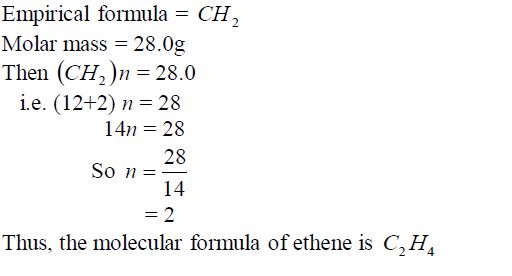Similarly, suppose carbon dioxide has a molar mass of 44g and its empirical formula is CO2. Its molecular formula can be determined thus: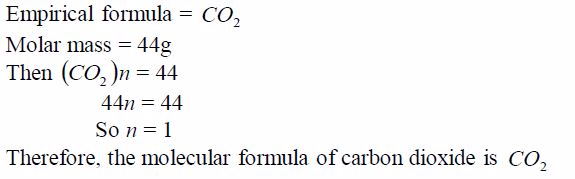The Empirical and Molecular Formulae
Calculate the empirical and molecular formulae
The Empirical Formula
Chemists find the percentage by mass of each element in a compound by experiment. Using this information, it is then possible to find the simplest formula of that compound. To do this we shall also need to know the relative atomic mass of each element present in the compound.
An experiment to find the empirical formula of a compound
To work out the empirical formula you need to know the masses of elements that combine. For example, magnesium combines with oxygen to form magnesium oxide. The masses that combine can be found like this:
Procedure
1. Weigh a crucible and lid, empty. Then add a coil of magnesium ribbon and weigh again.
2. Heat the crucible. Raise the lid carefully at intervals to let oxygen in. The magnesium ribbon burns brightly.
3. When burning is complete, let the crucible cool (still with its lid on). Then, weigh again. The increase in mass is due to oxygen.
Results
Here are sample results and the calculation:
• Mass of crucible + lid = 25.2 g
• Mass of crucible + lid + magnesium = 27.6g
• Mass of crucible + lid + magnesium oxide = 29.2g
• Mass of magnesium = 27.6g – 25.2g = 2.4g
• Mass of magnesium oxide = 29.2g – 25.2g = 4.0g
• Mass of oxygen, therefore = 4.0g – 2.4g =1.6g
Therefore, 2.4g of magnesium combines with 1.6g of oxygen.The RAMs are Mg = 24, O = 16. Changing masses to moles:24/2.4moles of magnesium atoms combines with1.6/16 moles of oxygen atoms
0.1 moles magnesium combines with 0.1moles of oxygen atoms.So the atoms combine in a ratio of 0.1:0.1 or simply 1:1The empirical formula of magnesium oxide = MgO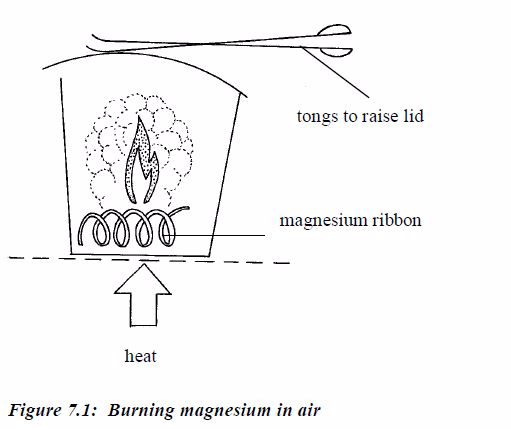Sometimes the empirical formula can be determined from the provided percentage composition or weight of atoms of the elements that constitute a compound.
Example 2
An experiment shows that 32g of sulphur combines with 32g ofoxygen to form the compound sulphur dioxide. What is itsempirical formula?
Solution
Divide each mass by the RAM of the respective element. This gives the ratio of different numbers of atoms of each element that make up the compound. Again, divide each of these ratios by the smallest to get the whole number ratio.
This gives the simplest ratio of the constituent elements possible. Sometimes you may not get a whole number ratio. If this happens, round off the ratio to the nearest whole number. Finally, write down the formula using the obtained ratio of the elements. Study the following table and make sure you understand the procedure: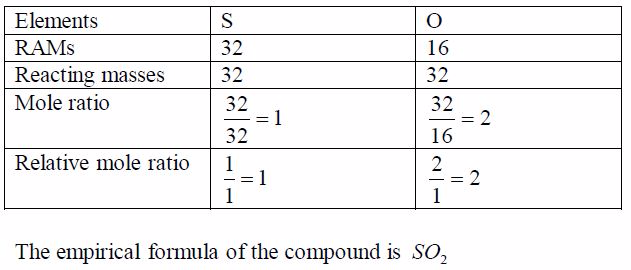Example 3
An oxide of carbon contains 27.3% carbon. Find the empirical formula of the oxide.Think big!What other element is present in the oxide of carbon?How do you know that the percentage of this other element is72.7%?
Solution
In order to work out the simplest formula we divide each percentage by the relative atomic mass of each element. This allows a comparison of the different numbers of atoms of each element that are present.
We get a ratio of each element with respect to each other as worked out in the table below. To get a whole number ratio, we again, divide each of these ratios by the smallest.The result shows the simplest ratio of atoms, in this case one carbon atom to two oxygen atoms. The simplest ratio, therefore,is CO2 .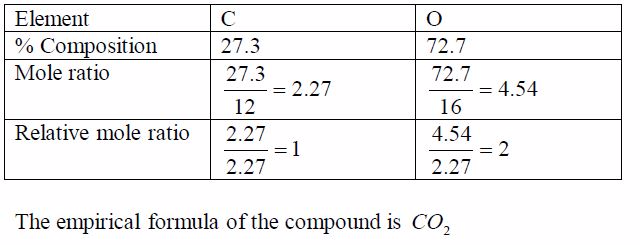Example 4
A compound X is a hydrocarbon. It contains only carbon and hydrogen atoms. 0.84g of X was completely burned in air. This produced 2.64g of carbon dioxide CO2 and 1.08g of water H2 O. Find the empirical formula of X.
Solution
1. In CO2 ,12/44of the mass is carbon
2. All the carbon came from X
So 2.64g of CO2 contains 2.64g〈12/44〉*2.64g= 0.72g of carbon and,therefore, 0.12g of hydrogen (0.84g – 0.72g = 0.12g)Since we have deduced the weights of the respective elements in the compound, we can now work out the empirical formula as usual: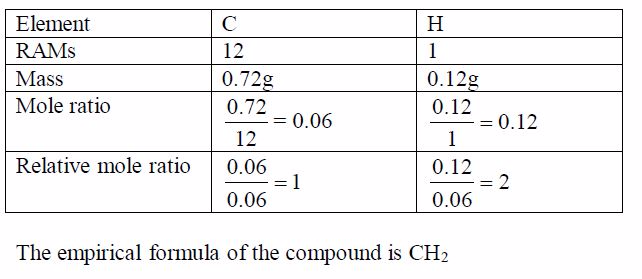The Molecular Formula
The molecular formula is more useful than the empirical formula because it gives more information. For some molecular compounds, both formulae are the same. For others they are different.
To find the molecular formula of an unknown compound youneed to know:
• the empirical formula; and
• the molecular weight of the compound.
• Once you have these two pieces of information you can now work out the molecular formula.
Example 5
A compound contains 85.7% carbon and 14.3% hydrogen. Itsmolecular weight is 28. What is its molecular formula?
Solution
Step 1: Find the empirical formula of the compound: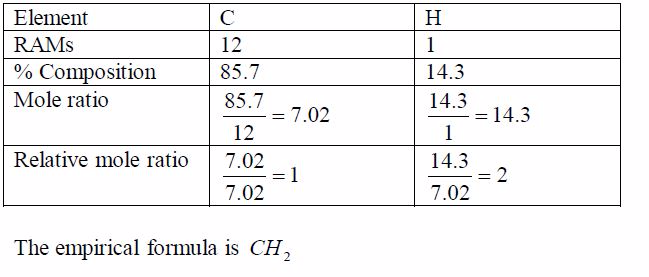Step 2: Find the molecular formula using the relation   2 CH n =Molecular formula: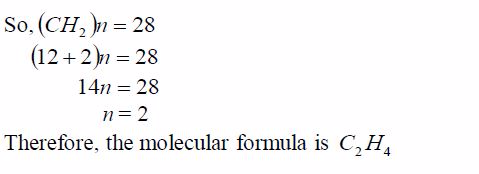Example 6
A compound contains 43.4% sodium, 11.3% carbon and 45.3%oxygen.
(a) Find the empirical formula of the compound.(b) If its molecular weight is 106, calculate its molecularformula.
Solution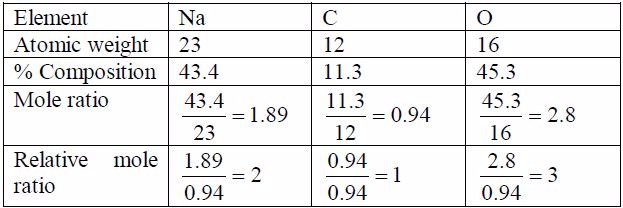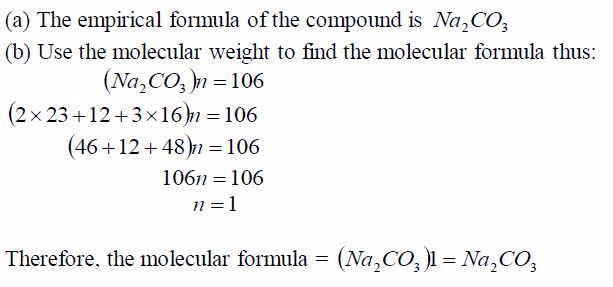In some cases, the molecular weights are given while in others the vapour density is given. To get the molecular weight,multiply the vapour density by two, e.g.:Molecular weight = Vapour density  2
Example 7
A hydrocarbon has a vapour density of 15, and it contains 20%by mass of hydrogen. Calculate the formula of the hydrocarbon.
Solution
If H = 20%, then C = 80%
Use these values to find the relative number of moles of atoms present: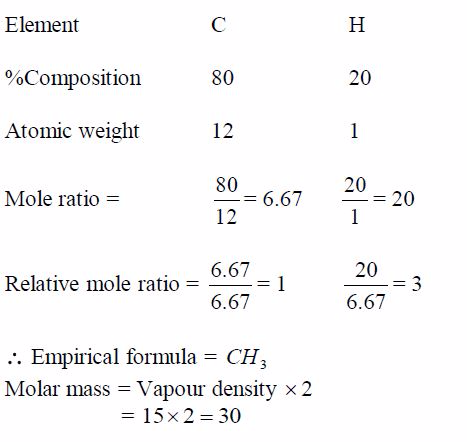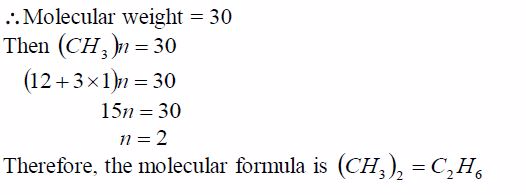The Concept of Oxidation State
Explain the concept of oxidation state
Oxidation state (or oxidation number) gives information about the number of electrons an element has lost, gained or shared on forming a compound. An element gains, loses or shares electron(s) only when it reacts to form a compound. An element in a free state has an oxidation state of zero.
There is a close correlation between valency and oxidation state.Oxidation state of an atom in a compound is normally assigned relative to the other elements in that particular compound. So you find that the oxidation state of a particular atom may change depending on a compound in which it is. For instance, the oxidation state of sulphur in SO2 is +4, whereas in SO3 is +6.
Rules for assigning oxidation numbers
Rule 1: An oxidation number of an element in a free(uncombined) state is zero e.g. Na, Li, K, Zn, etc.
Rule 2: Some elements nearly always have the same oxidation number in their compounds. These elements can be used as reference points in assigning oxidation numbers to the other elements. For example:
• Fluorine in all its compounds shows an oxidation number of -1.
• Chlorine in all its compounds has an oxidation number of -1 except when combined with fluorine or oxygen.
• Oxygen in all its compounds has an oxidation state of -2except in peroxides where it has an oxidation state of -1and oxygen difluoride (OF2) in which it has an oxidation state of +2.
• Hydrogen in all its compounds exhibits an oxidation state of +1 except in metal hydroxides where it shows an oxidation state of -1.
• Potassium in all its compounds exhibits an oxidation state of +1.
Rule 3: The algebraic sum of oxidation numbers of all elementsin a radical e.g. SO4 is equal to the charge possessed by theradical e.g. for the sulphate radical ( SO4 ) it is -2.
Rule 4: The algebraic sum of oxidation numbers in a neutralmolecule (or compound) is always equal to zero.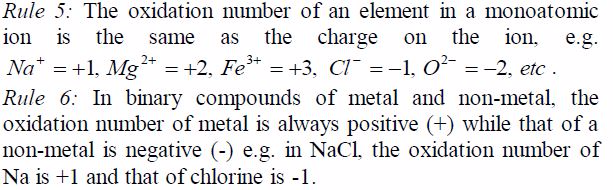Calculations of oxidation numbers
Example 8
Find the oxidation state of chlorine in the compound, KClO3
solution
The oxidation number of potassium, K = +1 and that of oxygen,O = —2. But since there are 3 oxygen atoms, we have 2*3 =—6.KClO3 is a neutral compound, so the sum of oxidation numbersof all elements in the compound is zero.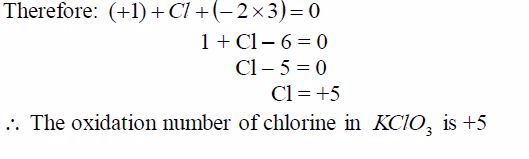Example 9
Find the oxidation state of sulphur in a molecule, H2 SO4
solution
The oxidation state of oxygen = -2The oxidation state of hydrogen = +1The sum of oxidation number of all elements in H2 SO4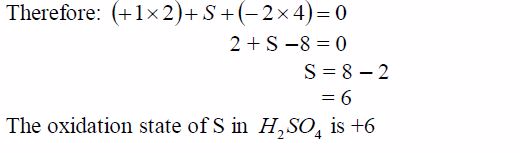Difference between Oxidation State and Valence
Differentiate oxidation state and valence
Valence electrons are the electrons that participate in forming chemical bonds. For example, lets look at the element carbon. Carbon has a total of 6 electrons (you can tell this by looking at the periodic table). However, 2 of those electrons are in the core of the atom ( in the 1s orbital). The remaining 4 electrons are in the outer 2s and 2p orbitals. Since these 4 electrons are in the outer shell, they can participate in bonding. Therefore since there’s 4 electrons that carbon can share, we say that carbon has a valency (or as you call it a valency number) of 4.
Oxidation state is a number used to designate how oxidized an atom is in a compound or molecule. It is the hypothetical charge an atom would have if all of its bonds were completely ionic (rather than covalent).
Really oxidation state is a book-keeping formalism that allows us to track what is being oxidized or reduced in a chemical reaction by comparing the oxidation states of the reactants to those of the products.Hope that helps.
A radical is a group of elements which behaves like a singleatom in forming compounds. Radicals do not exist alone. Theyare always found combined with metals. The valency of theradical is equal to the charge on it.
Table 7.6 shows the valenciesof different radicals. Examples of compounds in which theradicals can be found are also included in the table. All radicalsare charged. The only common positively charged radical isammonium radical. All these radicals except ammonium radical,which is positively charged, can combine with hydrogen or othermetals.
Table 7.6: Some radicals and their valencies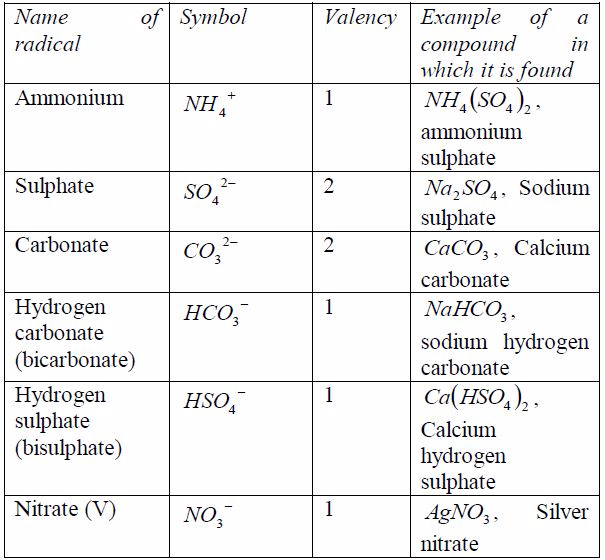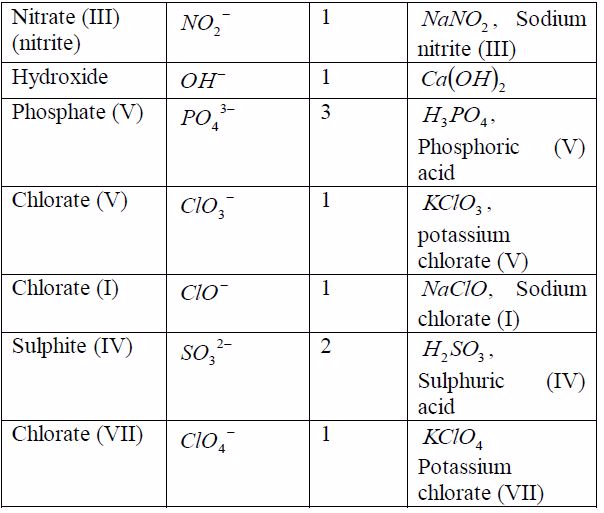As you have seen in the examples in the table above and in theprevious section, when writing the formula of any substance,you have to take into account the valencies of the reactingelements or radicals.
Example 10
Write the chemical formula of sodium sulphate
Solution
Step 1: Identify the elements or radicals in the compound:Sodium sulphate is a compound whose every molecule is madeup of a sodium metal and a sulphate radical.
Step 2: Write the symbols of the element and the radical; andtheir valencies: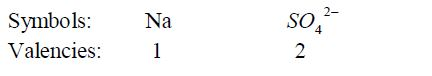Step 3: Interchange the valencies of the metal and radical andthen write down the formula.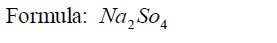Chemical Formula of Common Compounds
Write chemical formulae of common compounds
As you have seen in the examples in the table above and in the previous section, when writing the formula of any substance,you have to take into account the valencies of the reacting elements or radicals. the example is above

Covalent Bonding

The Concept of Covalent Bonding

Explain the concept of covalent bonding

Covalent bonding is a type of bonding which involves equalsharing of electrons between two or more atoms participating inbond formation. It is, generally, the property of non-metals to form covalent bonds. In a normal covalent bond, only electronsin the outermost shell of an atom are available for bondformation. Atoms share electrons so as to form a stable electronstructure of the nearby noble gas atom. Consider the bonding inthe following atoms:
Hydrogen
Hydrogen atom possesses only one electron. Its shell holds amaximum of 2 electrons, so it is not full. When two hydrogenatoms make a bond, each one donates one electron to the sharedpair to form a stable helium structure.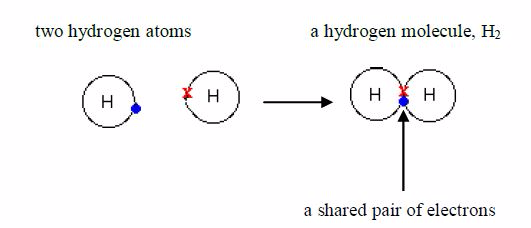Because atoms share electrons, there is a strong force ofattraction between them, holding them together. This force iscalled a covalent bond. The bonded atoms form a molecule.
Hydrogen gas is made up of hydrogen molecules and, for thisreason; it is called a molecular substance. Its formula is 2 HSeveral other non-metals are also molecular. For exampleChlorine, Cl2 , nitrogen, N2 iodine, I2 , oxygen O2 , sulphur,S8 , phosphorus, P4 , etc.
Chlorine
One molecule of chlorine is made up of two chlorine atoms.Each chlorine atom has an electronic configuration of 2:8:7. Itsconfiguration is only one electron less than the stable structurea shared pair of electrons of argon, 2,8,8. Each of the two chlorine atoms contributes oneelectron to the shared pair during chemical combination.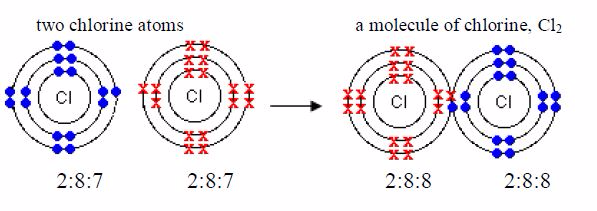Oxygen
The formula for oxygen is O2 . So each molecule must containtwo atoms. The electronic structure of oxygen is 2:6. Eachoxygen atom has only six outer electrons. So it needs two moreelectrons to reach a stable neon structure, 2:8. Therefore, eachatom contributes two electrons to be shared.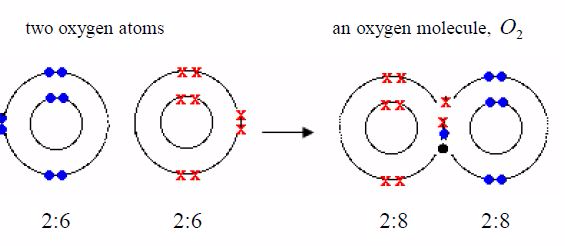Hydrogen chloride
The formula for hydrogen chloride is HCl. The electronicstructure for hydrogen atom is 1 and that for chlorine atom is2:8:7. An atom of hydrogen has 1 electron in its shell and achlorine atom has 7 outer electrons. To form a stable dupletstructure of helium, 2, the outer shell of hydrogen atom mustreceive one more electron and in order to form a stable argon structure, 2:8:8, the outer shell of chlorine atom must receiveone more electron. So, each atom must contribute one electronfor sharing.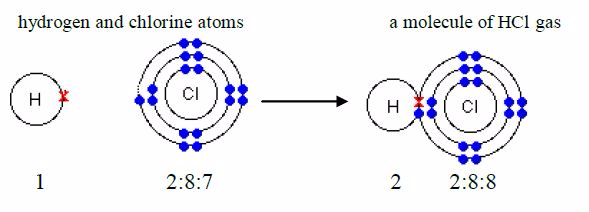In the above four examples, each hydrogen atom acquire thehelium structure (duplet), each chlorine atom achieves the argonconfiguration 2:8:8 (octet) and each oxygen atom acquires theneon configuration, 2:8 (octet).
It is possible to form multiple bonds between two non-metalatoms. When two electrons are shared between two atoms, onefrom each atom, we represent them by a single line, e.g. Cl-Cl.
When four electrons are shared such that each atom contributestwo electrons, we may represent the double bond formed by twolines, e.g. O=O. Likewise, when six electrons are shared suchthat each atom contributes three electrons, we may represent thetriple bond by three lines, N≡N.
Covalent Compounds
You have seen that several non-metal elements exist asmolecules. A huge number of compounds also exist asmolecules. In a molecular compound, atoms of differentelements share electrons with each other. These compounds areoften called electrovalent compounds because of the covalentbond in them.
Water, ammonia and methane are examples.Remember it was pointed early that only the electrons in theoutermost shells take part in bonding. As you can see in the examples below, only electrons in the outermost shells areinvolved in bond formation. Electrons in the inner shells do notnormally take part in bonding.
Water: Its formula is H O 2 . Each oxygen atom shares electronswith two hydrogen atoms.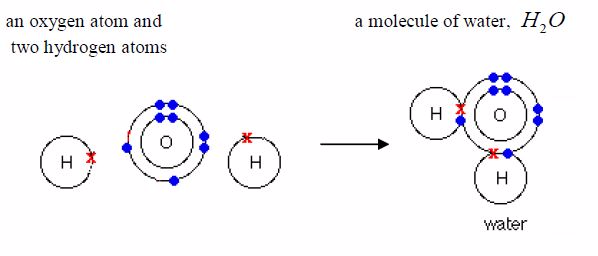Ammonia: Its formula is 3 NH . Each nitrogen atom shareselectrons with three hydrogen atoms.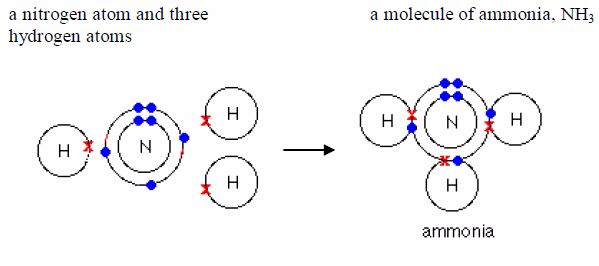Methane: Its formula is CH4. Each nitrogen atom shareselectrons with four hydrogen atoms.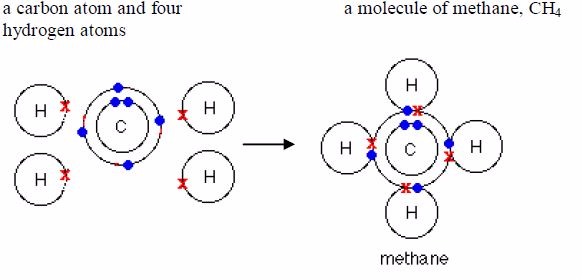The Properties of Covalent Bonding
State the properties of covalent bonding
Properties of covalent compounds
1. They are often liquids and gases at room temperature.
2. They have low melting and boiling points (low heats of fusion and vapourization).
3. They are usually soluble in organic solvents such as ethanol, ether, benzene, or carbon disulphide (very few are soluble in water).
4. They do not conduct electricity because they contain no ions and so are non-electrolytes. Electrovalent compounds consist of molecules.

The Concept of Electrovalent Bonding
Explain the concept of electrovalent bonding
This type of bonding occurs when an atom transfers electron(s) from its outermost shell to the outermost shell of another atom, usually a non-metal. In this type of bonding, an atom of a metallic element or group loses its valency electrons from its outermost shell. The lost electrons pass over to the outer shells of an atom with which the metal is combining.
By so doing, the metal will become positively charged due to excess proton(s) left on the nucleus while the non-metal will become negatively charged due to extra electron(s) it has received. The particles are then known as ions. The positive ions are called cations while the negative ions are called anions.
Metal atoms lose electrons to attain the electron configuration ofthe nearest noble gas, while the non-metal atoms gain electronsto attain the electron configuration of the nearest noble gas. Thismeans that an electron octet is left behind in the metal andcreated in the non-metal.
Let us consider the formation of the following compounds froma combination of metallic and non-metallic atoms:
Example 1: Sodium chloride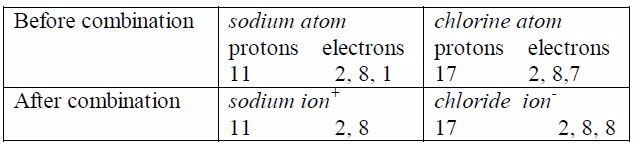Both ions now posses stable outer octets, like a noble gas.As you have seen, no molecule of sodium chloride is formed.Cations ( Na ) and anions Cl attract one another and arrangethemselves into a rigid, solid shape called a crystal, but theyremain quite separate. The combination can be expressed only inionic form as NaCl
The ionic compound is thus a cluster of ions in which a positiveion is surrounded spatially by a number of negative ions, while anumber of positive ions similarly surround each negative ion.The force holding together the oppositely charged ions is calledelectrostatic force and hence the name electrovalent bond.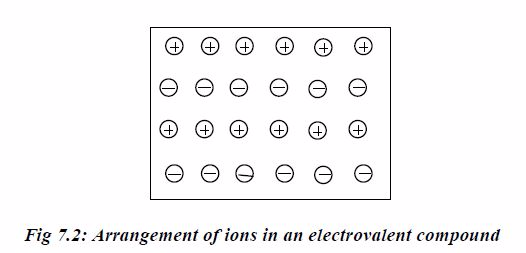Example 2: Calcium chloride
In the calcium ion, the two excess nuclear protons produce adouble positive charge, and the two electrons released from theouter shell of calcium atom are equally shared between the twochloride atoms. In each chloride ion, the excess electronproduces a single negative charge that is Ca 2Cl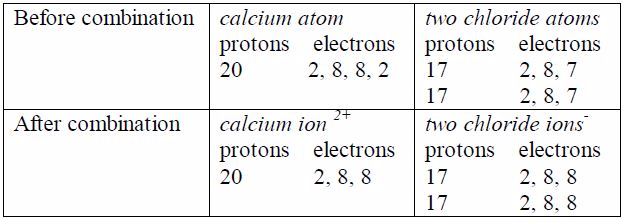Activity 1
Assignment
Magnesium oxide is an electrovalent compound just like calcium oxide and sodium chloride. Show how the magnesium atom combines with oxygen atom to form the oxide, clearly indicating the process of electron loss and gain
Properties of Electrovalent Bonding
State properties of electrovalent bonding
Properties of electrovalent (ionic) compounds
1. Ionic compounds are generally crystalline solids at room temperature.
2. They have high melting and boiling points (also high heats of fusion and vapourization)
3. They are generally soluble in water but insoluble in organic solvents such as benzene, alcohol and ether.
4. They conduct electricity when molten or when dissolved in water (not when solid).